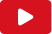Testo Names

Testo Names

Always on the [?]
All made of [?]
Names are good for friends so I
Don't need one yet

Always up in all the words
Keeping you in
Major round
Major round

Let me be here when the sun rises
Let me be here when the bells ring
Let me be here when the filthy rich catch fire

Let me be here when the sun rises
Let me be here when the bells ring
Let me be here when the filthy rich catch fire

Keepin' all the shudders down
To lower the risk
The future and it's golden doors
And I'm in for this
Always toxin, all the words
Keepin' it in
Major round
Major round

Let me be here when the sun rises
Let me be here when the bells ring
Let me be here when the filthy rich catch fire

Let me be here when the sun
Let me be here when the sun
Let me be here when the bells ring

Let me be here when the sun rises
Let me be here when the bells ring
Let me be here when the filthy rich catch fire

Guarda il video di NamesTesti Klangstof